Demo problem: Flow past a cylinder with a waving flag

In this example we consider the flow in a 2D channel that contains a cylinder with a waving "flag". This is a "warm-up problem" for the solution of Turek & Hron's FSI benchmark problem discussed in another tutorial.

# The Problem

The figure below shows a sketch of the problem: A 2D channel of height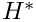and length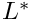contains a cylinder of diameter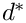, centred at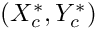to which a "flag" of thickness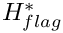and length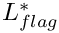is attached. We assume that the flag performs time-periodic oscillations with periodSteady Poiseuille flow with average velocity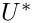is imposed at the left end of the channel while we assume the outflow to be parallel and axially traction-free.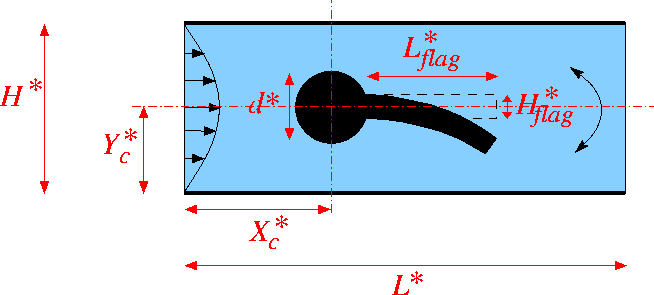Sketch of the problem in dimensional variables.

We non-dimensionalise all length and coordinates on the diameter of the cylinder,, time on the natural timescale of the flow,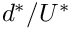, the velocities on the mean velocity,, and the pressure on the viscous scale. The problem is then governed by the non-dimensional Navier-Stokes equations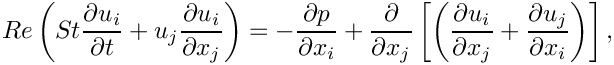where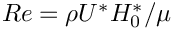and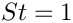, and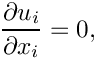subject to parabolic inflow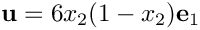at the inflow cross-section; parallel, axially-traction-free outflow at the outlet; and no-slip on the stationary channel walls and the surface of the cylinder,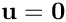. The no-slip condition on the moving flag iswhere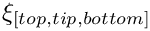are Lagrangian coordinates parametrising the three faces of the flag. The flag performs oscillations with non-dimensional period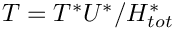. Here is a sketch of the non-dimensional version of the problem: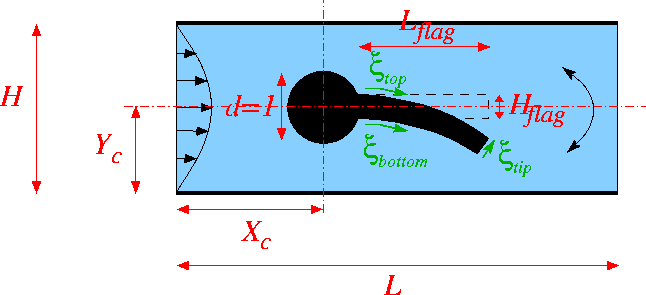Sketch of the problem in dimensionless variables, showing the Lagrangian coordinates that parametrise the three faces of the flag.

# Results

The figure below shows a snapshot of the flow field
(pressure contours and instantaneous streamlines) for a Reynolds number of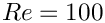and an oscillation period of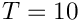, as well as the corresponding fluid mesh. Note how oomph-lib's automatic mesh adaptation has refined the mesh in the high-shear regions near the front of the cylinder and at the trailing edge of the flag.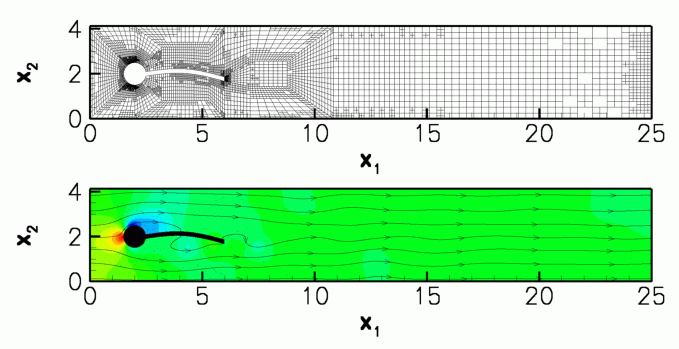Mesh (top) and flow field (bottom).

The corresponding animation illustrates the algebraic node update strategy (implemented with an AlgebraicMesh, discussed in more detail in another tutorial) and the evolution of the flow field. Note that the instantaneous streamlines intersect the (impermeable) flag because the flag is not stationary.

# The global parameters

As usual we use a namespace to define the (single) global parameter, the Reynolds number.

//====start_of_global_parameters==========================================
/// Global parameters
//========================================================================
{
/// Reynolds number
double Re=100.0;
}
Global parameters.
double Re
Reynolds number.

# Specification of the flag geometry

We specify the flag geometry and its time-dependent motion by representing its three faces by GeomObjects, each parametrised by its own Lagrangian coordinate, as shown in the sketch above. The geometry of the cylinder is represented by one of oomph-lib's generic GeomObjects, the Circle.

We enclose the relevant data in its own namespace and start by defining the period of the oscillation, and the geometric parameters controlling the flag's initial shape and its subsequent motion.

//====start_of_flag_definition===========================================
/// Namespace for definition of flag boundaries
//=======================================================================
namespace Flag_definition
{
/// Period of prescribed flag oscillation
double Period=10.0;
/// Height of flag
double H=0.2;
/// Length of flag
double L=3.5;
/// x position of centre of cylinder
double Centre_x=2.0;
/// y position of centre of cylinder
double Centre_y=2.0;
/// Amplitude of tip deflection
double Amplitude=0.33;
/// Pointer to the global time object
Time* Time_pt=0;
Namespace for definition of flag boundaries.
double Centre_y
y position of centre of cylinder
double Period
Period of prescribed flag oscillation.
double Centre_x
x position of centre of cylinder
double H
Height of flag.
double L
Length of flag.
double Amplitude
Amplitude of tip deflection.
Time * Time_pt
Pointer to the global time object.

We choose the motion of the flag such that it vaguely resembles that expected in the corresponding FSI problem: We subject the flag's upper and lower faces to purely vertical displacements that deform them into a fraction of a sine wave, while keeping the face at the tip of the flag straight and vertical. We implement this by prescribing the time-dependent motion of the two vertices at the tip of the flag with two functions:

/// Time-dependent vector to upper tip of the "flag"
Vector<double> upper_tip(const double& t)
{
double tmp_ampl=Amplitude;
if (t<=0.0) tmp_ampl=0.0;
Vector<double> uppertip(2);
uppertip= Centre_y+0.5*H-
tmp_ampl*sin(2.0*MathematicalConstants::Pi*t/Period);
return uppertip;
}
/// Time-dependent vector to bottom tip of the "flag"
Vector<double> lower_tip(const double& t)
{
double tmp_ampl=Amplitude;
if (t<=0.0) tmp_ampl=0.0;
Vector<double> lowertip(2);
lowertip= Centre_y-0.5*H-
tmp_ampl*sin(2.0*MathematicalConstants::Pi*t/Period);
return lowertip;
} // end of bottom tip
Vector< double > lower_tip(const double &t)
Time-dependent vector to bottom tip of the "flag".
Vector< double > upper_tip(const double &t)
Time-dependent vector to upper tip of the "flag".

This information is then used in three custom-written GeomObject that define the time-dependent shape of the three faces. Here is the one describing the shape of the upper face:

//-----start_of_top_of_flag--------------------------------------
/// GeomObject that defines the upper boundary of the flag
//---------------------------------------------------------------
class TopOfFlag : public GeomObject
{
public:
/// Constructor: It's a 1D object in 2D space
TopOfFlag() : GeomObject(1,2) {}
/// Destructor (empty)
~TopOfFlag(){}
/// Return the position along the top of the flag (xi varies
/// between 0 and Lx)
void position(const unsigned& t,const Vector<double> &xi, Vector<double> &r)
const
{
// Compute position of fixed point on the cylinder
Vector<double> point_on_circle(2);
point_on_circle=Centre_y+H/2.0;
r = point_on_circle+xi/L*(upper_tip(Time_pt->time(t))-
point_on_circle);
r = point_on_circle+xi/L*(upper_tip(Time_pt->time(t))-
point_on_circle)+
1.0/3.0*sin((r-point_on_circle)/
(upper_tip(Time_pt->time(t))-
point_on_circle)*MathematicalConstants::Pi)
*sin(2.0* MathematicalConstants::Pi*Time_pt->time(t)/Period);
}
/// Current position
void position(const Vector<double> &xi, Vector<double> &r) const
{
return position(0,xi,r);
}
/// Number of geometric Data in GeomObject: None.
unsigned ngeom_data() const {return 0;}
};

[We omit the definition of the other two GeomObjects, BottomOfFlag and TipOfFlag in in the interest of brevity. Their definitions can be found in the source code.] We provide storage for the pointers to the four GeomObjects required for the representation of the flag,

/// Pointer to GeomObject that bounds the upper edge of the flag
TopOfFlag* Top_flag_pt=0;
/// Pointer to GeomObject that bounds the bottom edge of the flag
BottomOfFlag* Bottom_flag_pt=0;
/// Pointer to GeomObject that bounds the tip edge of the flag
TipOfFlag* Tip_flag_pt=0;
/// Pointer to GeomObject of type Circle that defines the
/// central cylinder.
Circle* Cylinder_pt=0;
TipOfFlag * Tip_flag_pt
Pointer to GeomObject that bounds the tip edge of the flag.
Circle * Cylinder_pt
Pointer to GeomObject of type Circle that defines the central cylinder.
BottomOfFlag * Bottom_flag_pt
Pointer to GeomObject that bounds the bottom edge of the flag.
TopOfFlag * Top_flag_pt
Pointer to GeomObject that bounds the upper edge of the flag.

and provide a setup function that generates the GeomObjects and stores the pointer to the global Time object that will be created by the Problem:

/// Create all GeomObjects needed to define the cylinder and the flag
void setup(Time* time_pt)
{
// Assign pointer to global time object
Time_pt=time_pt;
// Create GeomObject of type Circle that defines the
// central cylinder.
/// Create GeomObject that bounds the upper edge of the flag
Top_flag_pt=new TopOfFlag;
/// Create GeomObject that bounds the bottom edge of the flag
Bottom_flag_pt=new BottomOfFlag;
/// Create GeomObject that bounds the tip edge of the flag
Tip_flag_pt=new TipOfFlag;
}
} // end of namespace that specifies the flag
void setup(Time *time_pt)
Create all GeomObjects needed to define the cylinder and the flag.

# The mesh

The figure below shows a sketch of the (unrefined) mesh and the enumeration of its boundaries. Various different implementations of the mesh exist, allowing the user to perform the node-update in response to the motion of the flag by a Domain/MacroElement - based procedure, or by using an algebraic node update. In either case, only the nodes in the elements that are shaded in yellow (or refined elements that are generated from these) participate in the node-update.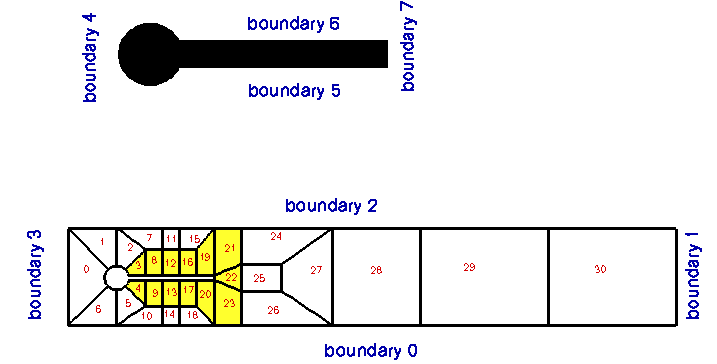The (unrefined) mesh. Only nodes in the yellow regions participate in the node-update.

The node update strategy is illustrated in the animation of the flow field and the mesh motion.

# The driver code

The driver code has the usual structure for a time-dependent problem: We store the command line arguments, create a DocInfo object, and assign the parameters that specify the dimensions of the channel.

//======start_of_main==================================================
/// Driver code -- pass a command line argument if you want to run
/// the code in validation mode where it only performs a few steps
//=====================================================================
int main(int argc, char* argv[])
{
// Store command line arguments
// Set up doc info
DocInfo doc_info;
doc_info.set_directory("RESLT");
doc_info.number()=0;
// Length and height of domain
double length=25.0;
double height=4.1;
int main(int argc, char *argv[])
//////////////////////////////////////////////////////////////////// ////////////////////////////////...

We build the problem using a mesh in which the node update is performed by the AlgebraicMesh class. (The driver code also provides the option to use a Domain/MacroElement - based node update – this option is chosen via suitable #ifdefs; see the source code for details).

// Create Problem with AlgebraicMesh-based node update
<AlgebraicElement<RefineableQCrouzeixRaviartElement<2> > >
problem(length, height);
///////////////////////////////////////////////////////////////// ///////////////////////////////////...

Next we set up the time-stepping (as usual, fewer timesteps are performed during a validation run which is identified by a non-zero number of command line arguments):

// Number of timesteps per period
unsigned nsteps_per_period=40;
// Number of periods
unsigned nperiod=3;
// Number of timesteps (reduced for validation)
unsigned nstep=nsteps_per_period*nperiod;
if (CommandLineArgs::Argc>1)
{
nstep=2;
// Also reduce the Reynolds number to reduce the mesh refinement
}
//Timestep:
double dt=Flag_definition::Period/double(nsteps_per_period);
/// Initialise timestep
problem.initialise_dt(dt);

We start the simulation with a steady solve, allowing up to three levels of adaptive refinement (fewer if we are performing a validation run):

// Solve adaptively with up to max_adapt rounds of refinement (fewer if
// run during self-test)
if (CommandLineArgs::Argc>1)
{
}
/// Output intial guess for steady Newton solve
problem.doc_solution(doc_info);
doc_info.number()++;

Finally, we enter the proper timestepping loop, allowing one spatial adaptation per timestep and suppressing the re-assignment of initial conditions following an adaptation by setting the parameter first to false. (See the discussion of timestepping with automatic mesh adaptation in another tutorial.)

// Do steady solve first -- this also sets the history values
// to those corresponding to an impulsive start from the
problem.doc_solution(doc_info);
doc_info.number()++;
/// Reduce the max number of adaptations for time-dependent simulation
// We don't want to re-assign the initial condition after the mesh
bool first=false;
// Timestepping loop
for (unsigned istep=0;istep<nstep;istep++)
{
// Solve the problem
// Output the solution
problem.doc_solution(doc_info);
// Step number
doc_info.number()++;
}
}//end of main

# The Problem class

The problem class provides an access function to the mesh (note the use of #ifdefs to choose the correct one), and defines the interfaces for the usual member functions, either empty or explained in more detail below.

//======= start_of_problem_class=====================================
/// Flow around a cylinder with flag
//===================================================================
template<class ELEMENT>
class TurekNonFSIProblem : public Problem
{
public:
/// Constructor: Pass length and height of domain.
TurekNonFSIProblem(const double &length,
const double &height);
/// Update the problem specs after solve (empty)
/// Update the problem specs before solve (empty)
/// After adaptation: Unpin pressures and pin redudant pressure dofs.
/// Update the velocity boundary condition on the flag
#ifdef USE_MACRO_ELEMENTS
/// Access function for the specific mesh
RefineableCylinderWithFlagMesh<ELEMENT>* mesh_pt()
{
return dynamic_cast<RefineableCylinderWithFlagMesh<ELEMENT>*>
(Problem::mesh_pt());
}
#else
/// Access function for the specific mesh
RefineableAlgebraicCylinderWithFlagMesh<ELEMENT>* mesh_pt()
{
return dynamic_cast<RefineableAlgebraicCylinderWithFlagMesh<ELEMENT>*>
(Problem::mesh_pt());
}
#endif
/// Doc the solution
void doc_solution(DocInfo& doc_info);
private:
/// Height of channel
double Height;
};//end_of_problem_class
void actions_after_newton_solve()
Update the problem specs after solve (empty)
void actions_before_implicit_timestep()
Update the velocity boundary condition on the flag.
void doc_solution(DocInfo &doc_info)
Doc the solution.
double Height
Height of channel.
After adaptation: Unpin pressures and pin redudant pressure dofs.
TurekNonFSIProblem(const double &length, const double &height)
Constructor: Pass length and height of domain.
void actions_before_newton_solve()
Update the problem specs before solve (empty)
RefineableCylinderWithFlagMesh< ELEMENT > * mesh_pt()
Access function for the specific mesh.

# The problem constructor

The initial assignment of zero velocity and pressure provides a very poor initial guess for the preliminary steady Newton solve. We therefore increase the maximum residuals and iteration counts allowed in the Newton iteration before creating the timestepper and setting up the GeomObjects required to parametrise the flag:

//=====start_of_constructor===============================================
/// Constructor: Pass length and height of domain.
//========================================================================
template<class ELEMENT>
const double &length, const double &height) : Height(height)
{
// Bump up Newton solver parameters to allow for crappy initial guesses
Max_residuals=100.0;;
Max_newton_iterations=50;
// Allocate the timestepper
// Setup flag/cylinder geometry

Next we build the mesh, passing the pointers to the various GeomObjects and the geometric parameters to its constructor.

// Build mesh (with AlgebraicMesh-based node update)
Problem::mesh_pt()=new RefineableAlgebraicCylinderWithFlagMesh<ELEMENT>
length, height,
time_stepper_pt());

We perform two rounds of uniform refinement (the Newton solver does not converge on coarser meshes) before creating an error estimator for the subsequent automatic mesh adaptation.

// Refine uniformly
mesh_pt()->refine_uniformly();
mesh_pt()->refine_uniformly();
// Set error estimator
Z2ErrorEstimator* error_estimator_pt=new Z2ErrorEstimator;
mesh_pt()->spatial_error_estimator_pt()=error_estimator_pt;

Both velocity components are imposed by Dirichlet conditions (either via a no-slip condition or via the imposed inflow profile) on all boundaries, apart from the outflow cross-section (mesh boundary 1), where the axial velocity is unknown.

// Set the boundary conditions for this problem: All nodes are
// free by default -- just pin the ones that have Dirichlet conditions
// here.
//Pin both velocities at all boundaries apart from outflow
unsigned num_bound = mesh_pt()->nboundary();
for(unsigned ibound=0;ibound<num_bound;ibound++)
{
unsigned num_nod= mesh_pt()->nboundary_node(ibound);
for (unsigned inod=0;inod<num_nod;inod++)
{
// Parallel, axially traction free outflow at downstream end
if (ibound != 1)
{
mesh_pt()->boundary_node_pt(ibound,inod)->pin(0);
mesh_pt()->boundary_node_pt(ibound,inod)->pin(1);
}
else
{
mesh_pt()->boundary_node_pt(ibound,inod)->pin(1);
}
}
} // done bc

Next we complete the build of the elements, passing the relevant pointers to physical parameters,

// Pass pointer to Reynolds number to elements
unsigned nelem=mesh_pt()->nelement();
for (unsigned e=0;e<nelem;e++)
{
// Upcast from GeneralisedElement to the present element
ELEMENT* el_pt = dynamic_cast<ELEMENT*>(mesh_pt()->element_pt(e));
//Set the Reynolds number
el_pt->re_pt() = &Global_Parameters::Re;
//Set the Womersley number (assuming St=1)
el_pt->re_st_pt() = &Global_Parameters::Re;
}

and impose the steady Poiseuille profile at the inlet (mesh boundary 3).

// Setup Poiseuille flow along boundary 3
unsigned ibound=3;
unsigned num_nod= mesh_pt()->nboundary_node(ibound);
for (unsigned inod=0;inod<num_nod;inod++)
{
double ycoord = mesh_pt()->boundary_node_pt(ibound,inod)->x(1);
// Set Poiseuille velocity
double uy = 6.0*ycoord/Height*(1.0-ycoord/Height);
mesh_pt()->boundary_node_pt(ibound,inod)->set_value(0,uy);
mesh_pt()->boundary_node_pt(ibound,inod)->set_value(1,0.0);
}

Finally, we pin the redundant pressure degrees of freedom (see another tutorial for details), and assign the equations numbers.

// Pin redudant pressure dofs
RefineableNavierStokesEquations<2>::
pin_redundant_nodal_pressures(mesh_pt()->element_pt());
// Assign equations numbers
cout <<"Number of equations: " << assign_eqn_numbers() << endl;
} // end of constructor

After each adaptation, we unpin and re-pin all redundant pressures degrees of freedom (see another tutorial for details). Since the inflow profile is parabolic, it is interpolated correctly from "father" to "son" elements during mesh refinement so no further action is required.

//========================================================================
template <class ELEMENT>
{
// Unpin all pressure dofs
RefineableNavierStokesEquations<2>::
unpin_all_pressure_dofs(mesh_pt()->element_pt());
// Pin redundant pressure dofs
RefineableNavierStokesEquations<2>::
pin_redundant_nodal_pressures(mesh_pt()->element_pt());

# Actions before the timestep

Before each timestep we update the nodal positions in the mesh and re-apply the no-slip condition at the nodes on mesh boundaries 5 - 7.

//==== start_of_actions_before_implicit_timestep==========================
/// Actions before implicit timestep: Update velocity boundary conditions
//========================================================================
template <class ELEMENT>
{
// Update the domain shape
mesh_pt()->node_update();
// Moving leaflet: No slip; this implies that the velocity needs
// to be updated in response to flag motion
for( unsigned ibound=5;ibound<8;ibound++)
{
unsigned num_nod=mesh_pt()->nboundary_node(ibound);
for (unsigned inod=0;inod<num_nod;inod++)
{
// Which node are we dealing with?
Node* node_pt=mesh_pt()->boundary_node_pt(ibound,inod);
// Apply no slip
FSI_functions::apply_no_slip_on_moving_wall(node_pt);
}
}
} //end_of_actions_before_implicit_timestep

# Post Processing

The function doc_solution(...) documents the results.

//==start_of_doc_solution=================================================
/// Doc the solution
//========================================================================
template<class ELEMENT>
{
ofstream some_file;
char filename;
// Number of plot points
unsigned npts;
npts=5;
// Output solution
sprintf(filename,"%s/soln%i.dat",doc_info.directory().c_str(),
doc_info.number());
some_file.open(filename);
mesh_pt()->output(some_file,npts);
some_file.close();
} // end_of_doc_solution

• Compare the results obtained when the node update is performed with the algebraic or the MacroElement/Domain-based node update. (oomph-lib's build machinery will automatically generate both versions of the code, using the -DUSE_MACRO_ELEMENTS compilation flag).

# Acknowledgements

• This code was originally developed by Stefan Kollmannsberger and his students Iason Papaioannou and
Orkun Oezkan Doenmez. It was completed by Floraine Cordier.